Courses

# Test: Hess's Law Of Constant Heat Summation

## 15 Questions MCQ Test Chemistry Class 11 | Test: Hess's Law Of Constant Heat Summation

Description
This mock test of Test: Hess's Law Of Constant Heat Summation for JEE helps you for every JEE entrance exam. This contains 15 Multiple Choice Questions for JEE Test: Hess's Law Of Constant Heat Summation (mcq) to study with solutions a complete question bank. The solved questions answers in this Test: Hess's Law Of Constant Heat Summation quiz give you a good mix of easy questions and tough questions. JEE students definitely take this Test: Hess's Law Of Constant Heat Summation exercise for a better result in the exam. You can find other Test: Hess's Law Of Constant Heat Summation extra questions, long questions & short questions for JEE on EduRev as well by searching above.
QUESTION: 1

### Direction (Q. Nos. 1-7) This section contains 7 multiple choice questions. Each question has four choices (a), (b), (c) and (d), out of which ONLY ONE option is correct. Given, The enthalpies of elements in their standard states are taken as zero. The enthalpy of formation of a compound

Solution:

Depend on whether the formation of compounds is exothermic or endothermic.For exothermic reaction, enthalpy of formation would be negative and for endothermic,it is  positive.

QUESTION: 2

### Given,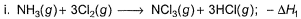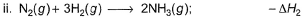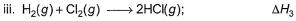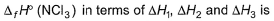Solution: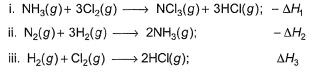Applying i-ii/2-3/2iii
We get ∆fH of NCl3 in terms of ∆H1, ∆H2 and ∆H3

QUESTION: 3

### Diborane is a potential rocket fuel which undergoes combustion according to the reaction,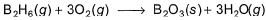From the following data, enthalpy change for the combustion of diborane is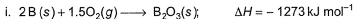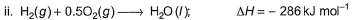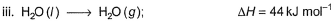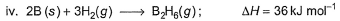Solution:

On applying equation 1 + 3 times equation 2 minus equation 4 plus equation 3, we will get enthalpy change for the combustion of diborane.

QUESTION: 4

Following diagram represents Born-Haber cycle to determine lattice energy of NaCI(s). It is based on Hess’s law of constant heat summation. ΔlatticeH° of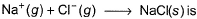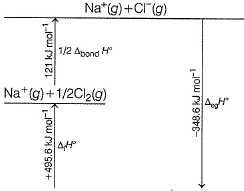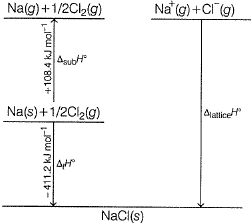Solution:

The correct answer is Option A.

-411.2 = 108.4 + 495.6 + 121.0 - 348.6 + LE
=>LE= -411.2 - 108.4 - 495.6 - 121.0 +348.6
=> LE = -787.6 ~ -788 kJ mol-1

QUESTION: 5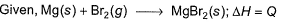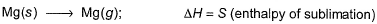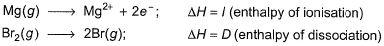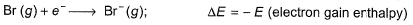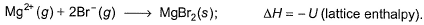Thus,

Solution:

Put the things we need as reactant on LHS the product on RHS. To do that, we will apply equation S+I+D-2E-U.

QUESTION: 6

Given, HCI (g) → H (g) + Cl (g) at 298 K (say temperature T K),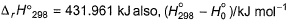For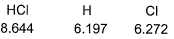Q. Thus, for the given reaction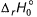(at 0 K) is

Solution:

Ho= Ho of product - Ho of reactant
so ho =(6.197 + 6.272 ) - 8.644
= 3.825
Ho298-Ho = 431.961 - 3.825=428.136532873

QUESTION: 7

The measured enthalpy change for burning of ketene (g) (CH2CO) is - 981.1 kJ mo-1 at 298 K ,CH2CO (g)+ 2O2(g) → 2CO2 (g) + H2O (g) and that of CH4 (g) is - 802.3 kJ mol-1 at 298 K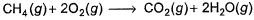Thus, enthalpy change at 298 K for the following thermochemical reaction is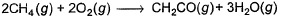Solution:

CH2CO (g)+ 2O2(g) → 2CO2 (g) + H2O (g)     -(i)
CH4 (g)+ 2O2(g) → CO2 (g) + 2H2O (g)     -(ii)

On 2(ii)-(i)

2CH4 (g)+ 2O2(g) → CH2CO (g) + 3H2O (g)     -(iii)
∆H(iii) = 2∆H(ii) - ∆H(i) = [2 (-802.3) -(-981.1)] -623.5 kJ mol-1

QUESTION: 8

Direction (Q. Nos. 8) This sectionis based on statement I and Statement II. Select the correct answer from the code given below.

Q. In the following thermochemical reactions,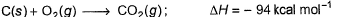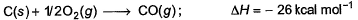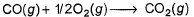Statement I : Enthalpy of combustion of CO(g) is - 26 kcal mol-1.

Statement II : CO2(g) is an exothermic compound.

Solution:

According to me, the question is presented in an improper way. For statement I to be correct, value of enthalpy of combustion of CO be -68 kcal mol-1. This can be sensed by reversing reaction II adding it to reaction I. By this, we will get the value of enthalpy of combustion of CO be -68 kcal mol-1
However, statement II is wrong. There is no such exothermic or endothermic compounds. Reactions are exothermic and not the products.

QUESTION: 9

Direction (Q. Nos. 9 and 10) This section contains a paragraph, wach describing  theory, experiments, data etc. three Questions related to paragraph have been  given.Each question have only one correct answer among the four given  ptions  (a),(b),(c),(d).

Consider the following thermochemical equations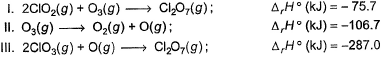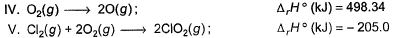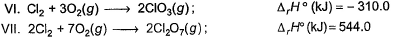Q. ΔrH° of the following thermochemical reaction is

CIO2 (g) + O (g)  CIO3 (g)

Solution:

The question can be simply done by understanding that this reaction is endothermic so ∆H is negative.

Hence A is correct.

QUESTION: 10

Consider the following thermochemical equationsQ. ΔrH° of the following thermochemical reaction is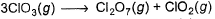Solution:
QUESTION: 11

Direction (Q. Nos. 11) Choice the correct combination of elements and column I and coloumn II  are given as option (a), (b), (c) and (d), out of which ONE option is correct.

Q. For liquid water at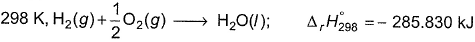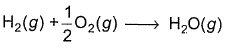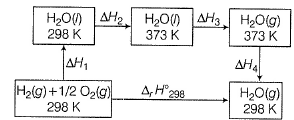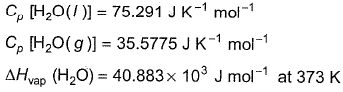Compare the parameters ΔH1, ΔH2 ... in Column I with the corresponding values in kJ in Column II.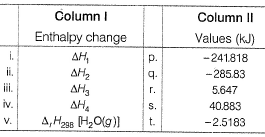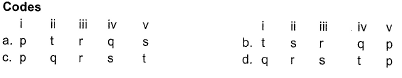Solution:

∆H1=∆rH298=-285.83kJmol-¹
∆H²=Cp(liq)∆T=75.29×75 =5.64kJmol-¹
∆H³=∆Hvap =40.88kJmol-¹
∆H⁴=Cp(gas)∆T=35.57×(-75) =-2.5183kJmol-¹

*Answer can only contain numeric values
QUESTION: 12

Direction (Q. Nos. 12-15) This section contains 4 questions. when worked out will result in an integer from 0 to 9 (both inclusive).

Q. Based on (BE) values, ΔrH° of the following reaction 298 K is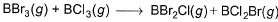Solution:

Let the BE value of B---Br bond be x and B---Cl bond be y
BE of BBr3 is 3x, BCl3=3y , BBr2Cl=2x+y ,BCl2Br=2y+x
then ∆Hr=Hr(products)-Hr(reactants)=(2x+y+2y+x) - (3x+3y)=0

*Answer can only contain numeric values
QUESTION: 13

Heat of combustion of CH2CO(g) is - 981.1 kJ mol-1 and that of CH4 (g) is - 802.3 kJat 298 K. If 1247.0 kJ of heat is released in the following change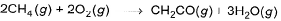Q. How many moles of CH4(g)are used?

Solution:

[2CH4 + 2O2 → CH2O + H2O]n
∆HC = n[-981.1-(-8023×2)]
1247       = n[-981.1+1604.6]
N[623.5] = 1247
N = 4
So, no of moles of CH4 = n×2 = 2×2 = 4

*Answer can only contain numeric values
QUESTION: 14

Consider the following reactions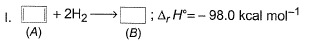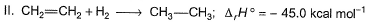Q. What is the resonance energy (in kcal) of A?

Solution:

Resonance energy = (Observed ∆H) - (Theoretical ∆H)
= (-98.0) - 2(-45.0)
= -8 kcal mol-1
So we have Resnance energy = 8

*Answer can only contain numeric values
QUESTION: 15

Given, enthalpy of combustion of carbon (s) = -94 kcal mol-1

H2(g) = - 68 kcal mol-1 and CXH4 = - 318 kcal mol-1 and  ΔfH° (CxH4) = - 100 kcal mol-1

Q. What is the value of x?

Solution: